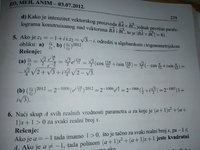# A little Complex question?

#### dolina dahani

##### New member
Hi there, I'm sorry if I made a mistake and put the thread in the wrong place. I have just a little question, I know how to do it but I'm just wondering something. So the question asks to find what Z1/Z2 is and (Z1/Z2)^2012 in algebraic and trigonometric form yet as a result they use exponential and then they transform it in trigonometric form. Am I missing something? There's also an almost the same question with the same answer. I know how to do it in trigonometric and exponential form but I have no clue how to do it in algebraic... The 5th question#### pka

##### Elite Member
$$z_1=-1+i=\sqrt{2}\exp\left(\dfrac{3\pi i}{4}\right)$$ and $$z_2=\sqrt3-i=2\exp\left(\dfrac{-\pi i}{6}\right)$$

We use the polar form for large powers for ease of computation

•topsquark

#### Dr.Peterson

##### Elite Member
If (I don't know your language) they are asking for the result "in algebraic and trigonometric form", that doesn't mean they require you to do the work in a particular form. You do what's easiest, and then give them the form they want. They're teaching you to think that way. (I suppose "algebraic form" means 'a + bi".)

#### pka

##### Elite Member
If (I don't know your language) they are asking for the result "in algebraic and trigonometric form", that doesn't mean they require you to do the work in a particular form. You do what's easiest, and then give them the form they want. They're teaching you to think that way. (I suppose "algebraic form" means 'a + bi".)
In my experience (western mathematics) there are two forms of a complex number, the rectangular and the polar form.
I suspect that algebraic, $$a+bi$$, forms are what we know as the rectangular form.
Also, what we call the polar form is also known as trigonometric form.
I have this standard post:
$$\arg(x + yi) = \left\{ {\begin{array}{{rl}} {\arctan \left( {\frac{y}{x}} \right),}&{x > 0} \\ {\arctan \left( {\frac{y}{x}} \right) + \pi ,}&{x < 0\;\& \;y > 0} \\ {\arctan \left( {\frac{y}{x}} \right) - \pi ,}&{x < 0\;\& \;y < 0} \end{array}} \right.$$

•topsquark